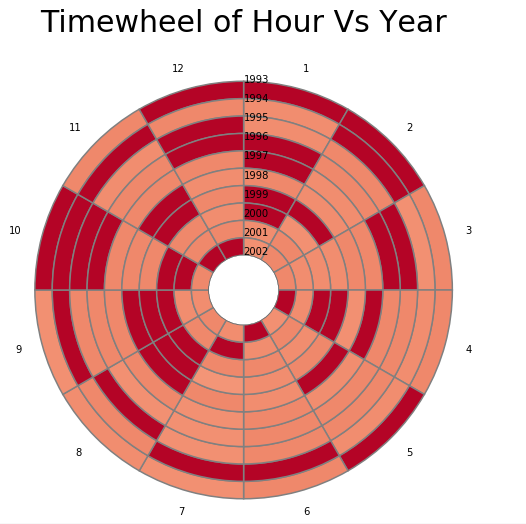﻿ Pandas Datetime: Timewheel of Hour Vs Year comparison of the top 10 years in which the UFO was sighted - w3resource

# Pandas Datetime: Timewheel of Hour Vs Year comparison of the top 10 years in which the UFO was sighted

## Pandas Datetime: Exercise-25 with Solution

Write a Pandas program to create a Timewheel of Hour Vs Year comparison of the top 10 years in which the UFO was sighted.

Sample Solution:

Python Code:

``````import pandas as pd
import matplotlib.pyplot as plt
import matplotlib as mpl
import matplotlib.cm as cm
#Source: https://bit.ly/2XDY2XN
df['Date_time'] = df['Date_time'].astype('datetime64[ns]')
def is_top_years(year):
if year in most_sightings_years.index:
return year
month_vs_year = df.pivot_table(columns=df['Date_time'].dt.month,index=df['Date_time'].dt.year.apply(is_top_years),aggfunc='count',values='city')
month_vs_year.index = month_vs_year.index.astype(int)
month_vs_year.columns = month_vs_year.columns.astype(int)
print("\nComparison of the top 10 years in which the UFO was sighted vs each month:")
def pie_heatmap(table, cmap='coolwarm_r', vmin=None, vmax=None,inner_r=0.25, pie_args={}):
n, m = table.shape
vmin= table.min().min() if vmin is None else vmin
vmax= table.max().max() if vmax is None else vmax

centre_circle = plt.Circle((0,0),inner_r,edgecolor='black',facecolor='white',fill=True,linewidth=0.25)
norm = mpl.colors.Normalize(vmin=vmin, vmax=vmax)
cmapper = cm.ScalarMappable(norm=norm, cmap=cmap)

for i, (row_name, row) in enumerate(table.iterrows()):
labels = None if i > 0 else table.columns
wedges = plt.pie( * m,radius=inner_r+float(n-i)/n, colors=[cmapper.to_rgba(x) for x in row.values],
labels=labels, startangle=90, counterclock=False, wedgeprops={'linewidth':-1}, **pie_args)
plt.setp(wedges, edgecolor='grey',linewidth=1.5)
wedges = plt.pie(, radius=inner_r+float(n-i-1)/n, colors=['w'], labels=[row_name], startangle=-90, wedgeprops={'linewidth':0})
plt.setp(wedges, edgecolor='grey',linewidth=1.5)
plt.figure(figsize=(8,8))
plt.title("Timewheel of Hour Vs Year",y=1.08,fontsize=30)
pie_heatmap(month_vs_year, vmin=-20,vmax=80,inner_r=0.2)
``````

Sample Output:

```Comparison of the top 10 years in which the UFO was sighted vs each month:
C:\Users\User\Anaconda3\lib\site-packages\matplotlib\colors.py:512: RuntimeWarning: invalid value encountered in less
xa[xa < 0] = -1```

Python Code Editor:

Have another way to solve this solution? Contribute your code (and comments) through Disqus.

What is the difficulty level of this exercise?

﻿

## Python: Tips of the Day

F strings:

It is a common practice to add variables inside strings. F strings are by far the coolest way of doing it. To appreciate the f strings more, let's first perform the operation with the format function.

```name = 'Owen'
age = 25
print("{} is {} years old".format(name, age))
```

Output:

```Owen is 25 years old
```

We specify the variables that go inside the curly braces by using the format function at the end. F strings allow for specifying the variables inside the string.

```name = 'Owen'
age = 25
print(f"{name} is {age} years old")
```

Output:

```Owen is 25 years old
```

F strings are easier to follow and type. Moreover, they make the code more readable.

```A, B, C = {2, 4, 6}
print(A, B, C)
A, B, C = ['p', 'q', 'r']
print(A, B, C)
```

Output:

```2 4 6
p q r
```